# GcsSloop

Just do IT later.

## Matrix简介

Matrix是一个矩阵，主要功能是坐标映射，数值转换。

Matrix作用就是坐标映射，那么为什么需要Matrix呢? 举一个简单的例子: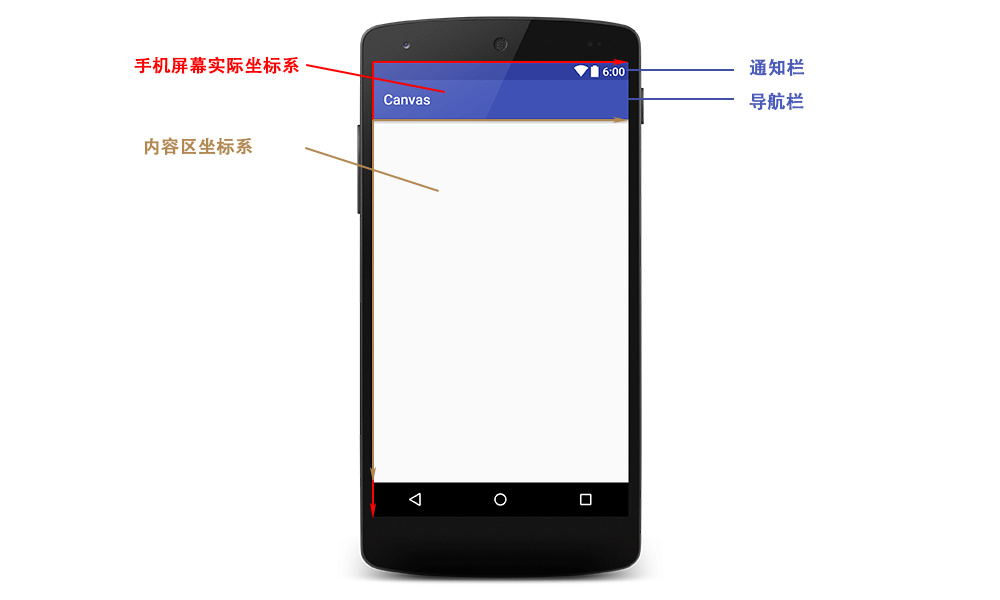### Matrix特点

• 作用范围更广，Matrix在View，图片，动画效果等各个方面均有运用，相比与之前讲解等画布操作应用范围更广。

• 更加灵活，画布操作是对Matrix的封装，Matrix作为更接近底层的东西，必然要比画布操作更加灵活。

• 封装很好，Matrix本身对各个方法就做了很好的封装，让开发者可以很方便的操作Matrix。

• 难以深入理解，很难理解中各个数值的意义，以及操作规律，如果不了解矩阵，也很难理解前乘，后乘。

### 常见误解

1.认为Matrix最下面的一行的三个参数(MPERSP_0、MPERSP_1、MPERSP_2)没有什么太大的作用，在这里只是为了凑数。

2.最后一个参数MPERSP_2被解释为scale

## Matrix基本原理

Matrix 是一个矩阵，最根本的作用就是坐标转换，下面我们就看看几种常见变换的原理: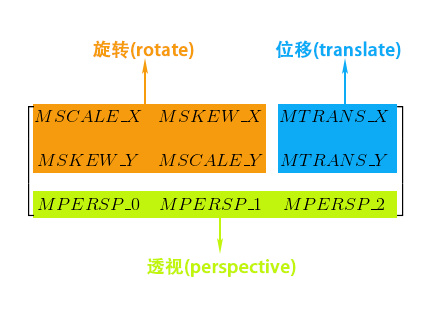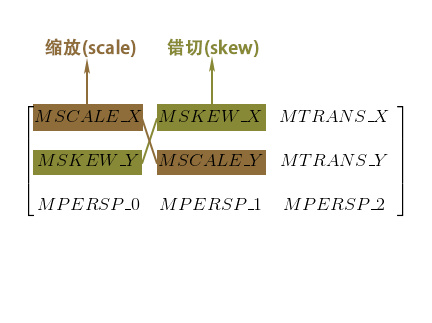### 1.缩放(Scale)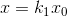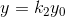(x, y, 1) - 点
(x, y, 0) - 向量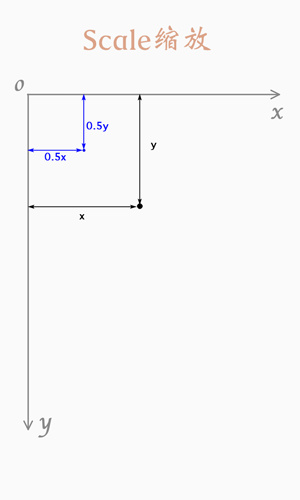### 2.错切(Skew)

#### 水平错切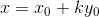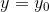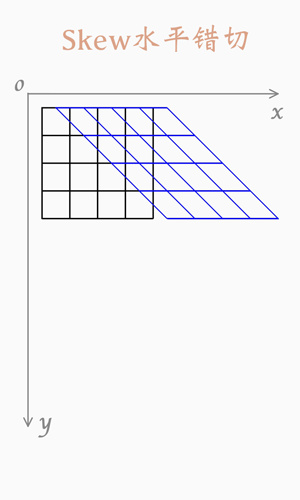#### 垂直错切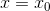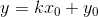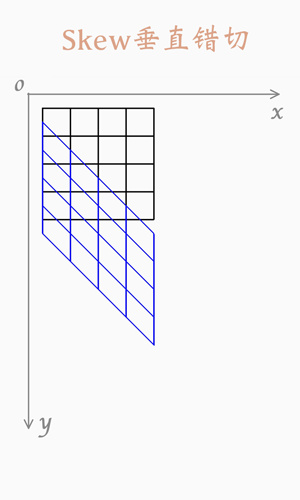#### 复合错切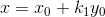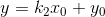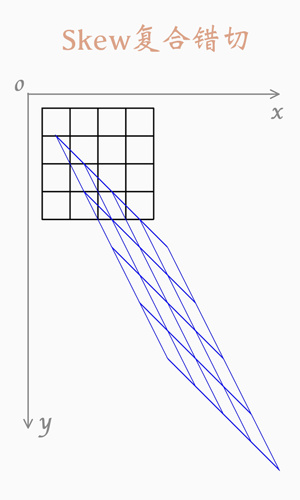### 3.旋转(Rotate)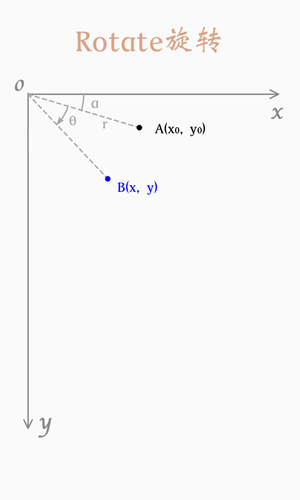### 4.平移(Translate)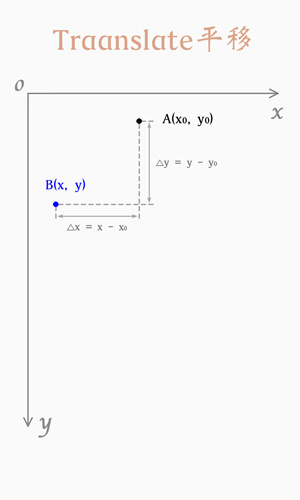## 组合

### 错误结论一：pre 是顺序执行，post 是逆序执行。

// 第一段 pre  顺序执行，先平移(T)后旋转(R)
Matrix matrix = new Matrix();
matrix.preTranslate(pivotX,pivotY);
matrix.preRotate(angle);
Log.e("Matrix", matrix.toShortString());

// 第二段 post 逆序执行，先平移(T)后旋转(R)
Matrix matrix = new Matrix();
matrix.postRotate(angle);
matrix.postTranslate(pivotX,pivotY)
Log.e("Matrix", matrix.toShortString());


• 矩阵乘法不满足交换律，即 A*B ≠ B*A
• 矩阵乘法满足结合律，即 (A*B)*C = A*(B*C)
• 矩阵与单位矩阵相乘结果不变，即 A * I = A
由于上面例子中原始矩阵(M)是一个单位矩阵(I)，所以可得：

// 第一段 pre
M' = (M*T)*R = I*T*R = T*R

// 第二段 post
M' = T*(R*M) = T*R*I = T*R


### 错误结论二：pre 是先执行，而 post 是后执行。

pre  ：先，和 before 相似。
post ：后，和 after  相似。


matrix.preRotate(angle);
matrix.postTranslate(pivotX,pivotY);


// 矩阵乘法满足结合律
M‘ = T*(M*R) = T*M*R = (T*M)*R


(这段内容注定要成为我写作历程中不可抹灭的耻辱，既然是公开文章，就应该对读者负责，虽然我在发表每一篇文章之前都竭力的求证其中的问题，各种细节，避免出现这种错误，但终究还是留下了这样一段内容，在此我诚挚的向我所有的读者道歉。)

## 以下是错误证明：

Matrix matrix = new Matrix();
matrix.postScale(0.5f, 0.8f);
matrix.preTranslate(1000, 1000);
Log.e(TAG, "MatrixTest" + matrix.toShortString());


Matrix matrix = new Matrix();
matrix.preScale(0.5f, 0.8f);
matrix.preTranslate(1000, 1000);
Log.e(TAG, "MatrixTest" + matrix.toShortString());


## 如何理解和使用 pre 和 post ？

pre  : 右乘， M‘ = M*A
post : 左乘， M’ = A*M


• 所有的操作(旋转、平移、缩放、错切)默认都是以坐标原点为基准点的。

• 之前操作的坐标系状态会保留，并且影响到后续状态。

1. 先将坐标系原点移动到指定位置，使用平移 T
2. 对坐标系进行旋转，使用旋转 S (围绕原点旋转)
3. 再将坐标系平移回原来位置，使用平移 -T


M 为原始矩阵，是一个单位矩阵， M‘ 为结果矩阵， T 为平移， R为旋转

M' = M*T*R*-T = T*R*-T


Matrix matrix = new Matrix();
matrix.preTranslate(pivotX,pivotY);
matrix.preRotate(angle);
matrix.preTranslate(-pivotX, -pivotY);


Matrix matrix = new Matrix();
matrix.preTranslate(pivotX,pivotY);
// 各种操作，旋转，缩放，错切等，可以执行多次。
matrix.preTranslate(-pivotX, -pivotY);


M' = M*T* ... *-T = T* ... *-T


Matrix matrix = new Matrix();
// 各种操作，旋转，缩放，错切等，可以执行多次。
matrix.postTranslate(pivotX,pivotY);
matrix.preTranslate(-pivotX, -pivotY);


M' = T*M* ... *-T = T* ... *-T


### 下面我们用不同对方式来构造一个相同的矩阵:

• 1.由于矩阵乘法不满足交换律，请保证使用初始矩阵(Initial Matrix)，否则可能导致运算结果不同。
• 2.注意构造顺序，顺序是会影响结果的。
• 3.Initial Matrix是指new出来的新矩阵，或者reset后的矩阵，是一个单位矩阵。

#### 1.仅用pre：

// 使用pre， M' = M*T*S = T*S
Matrix m ＝ new Matrix();
m.reset();
m.preTranslate(tx, ty);
m.preScale(sx, sy);


#### 2.仅用post:

// 使用post， M‘ = T*S*M = T*S
Matrix m ＝ new Matrix();
m.reset();
m.postScale(sx, sy);  //，越靠前越先执行。
m.postTranslate(tx, ty);


#### 3.混合:

// 混合 M‘ = T*M*S = T*S
Matrix m ＝ new Matrix();
m.reset();
m.preScale(sx, sy);
m.postTranslate(tx, ty);


// 混合 M‘ = T*M*S = T*S
Matrix m ＝ new Matrix();
m.reset();
m.postTranslate(tx, ty);
m.preScale(sx, sy);


Tips

CustomView